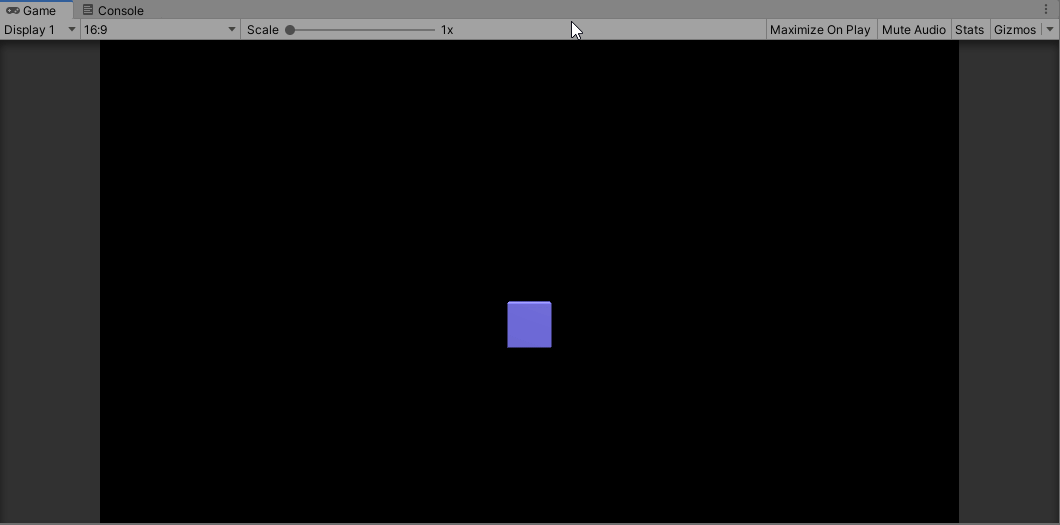# Problem Statement :

You have been given a number of stairs. Initially, you are at the 0th stair, and you need to reach the Nth stair. Each time you can either climb one step or two steps. You are supposed to return the number of distinct ways in which you can climb from the 0th step to Nth step.

Sample Input:

`3`

Sample Output:

`3`

# Explanation of given Test Case :

For N = 3

We can climb one step at a time i.e. {(0, 1) ,(1, 2),(2,3)} or we can climb the first two-step and then one step i.e. {(0,2),(1, 3)} or we can climb first one step and then two step i.e. {(0,1), (1,3)}.

# Matrix multiplication

If we were using “dp” it would take O(N) space. But there was no need of taking a space of O(N). Because if we look at any step of dp:

`dp[ currStep ] = dp[ currStep-1 ] + dp[ currStep-2 ]`

We only need the answer of the two-step and the one-step before the “currStep” for evaluation of the “currStep”. So we can replace “dp” with two variables let’s say “oneStep” and “twoSteps”. Where “oneStep” denotes the total number of ways to reach (currStep-1)th step from beginning and “twoSteps” denotes the total number of ways to reach (currStep-2)th step from the beginning. So,

`currStep=oneStep+twoStep`

So the above statement is (N-1)th Fibonacci Number. We can calculate the ‘N’th Fibonacci Number using Binary Matrix Exponentiation explained below. Here, Fn represents the nth Fibonacci number. We will raise the matrix to the power of (n — 1) so that the top-left element gives the value of F(n + 1–1) = F(n).

[[1 1] (pow n) [[(F(n+1) F(n)]

[1 0]] = [ F(n) F(n-1)]]

1. So we need to find the (N — 1)th Power of this Matrix.
2. We can write a function for the multiplication of two matrices.
3. Then we can use binary exponentiation to calculate the ’N’ power of this Matrix in log(N).
4. Binary Exponentiation / Fast Exponentiation:
`long long binpow(Matrix a, long long b) {if (b == 0)     return 1;Matrix res = binpow(a, b / 2);if (b % 2)     return res * res * a;else     return res * res;}`
1. Similarly, we can write a version for Matrix Exponentiation.
2. Take care of overflow using modulo by 10⁹ + 7 at each operation of Matrix Multiplication.

Time Complexity : O(log(N))
Space Complexity : O(log(N))

# Code :

Placewit grows the best engineers by providing an interactive classroom experience and by helping them develop their skills and get placed in amazing companies.

--

--

--

## More from Placewit

Upskilling students for tech placements!

Love podcasts or audiobooks? Learn on the go with our new app.

## Cooldown System in Unity## Code to create pattern in java## Beginning of beta testing of the plugin for Rider and PVS-Studio C# for Linux / macOS## The Difference between _() and {% trans %} in Django Templates## Twitter Stock Market Sentiment Analysis## Knowing how to create algorithms will be a fundament skill by 2035## Placewit

Upskilling students for tech placements!

## Longest Increasing Subsequence Coding Question## Interview Questions For Developers To Ask Potential Employers## Validate Stack Sequences ( Daily Leetcode )